Ever wonder how to tell what columns would be good for indexes? Once the index is created, ever wonder why you never see it in SQL explain plans you would expect to see it in???

There are 2 useful statistics that can be used to answer both of these questions.

Lets look at column density first.

In TOAD, do a F4/Describe of the EMP table and you will see this on the columns statistics.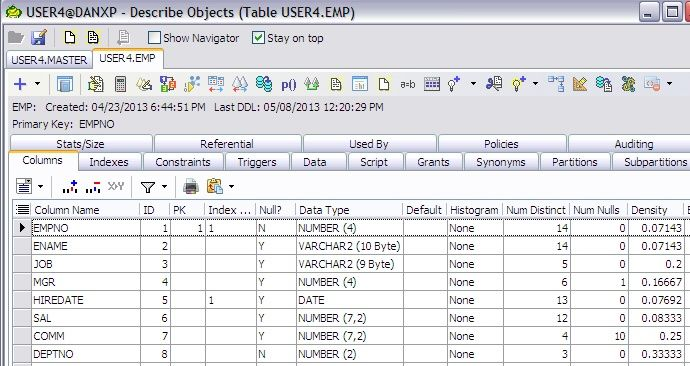You can easily see all the columns in the EMP table and various statistics.

We can see:

1. That there are no histograms
2. The number of distinct values
3. The column value density across all of the rows (how often percentage wise does any data value appear)

The closer the density is to 0, the more likely Oracle will use such an index instead of a full-table scan. We can see, for example, that the HIREDATE would be a good column to index.

Looking at another important statistic on the Indexes tab…after the index is create…how likely is Oracle to actually USE the index when the indexed column appears in a SQL where clause? This statistic is clustering factor.

Clustering Factor is the relationship of the index leaf blocks and how many DIFFERENT table blocks each leaf block points to.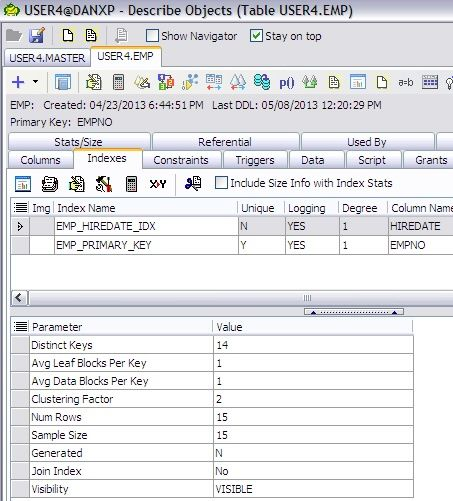We can see the EMP_HIREDATE_IDX has a number of useful statistics including both Clustering Factor and Number of Rows. I generally like to compare clustering factor to the number of blocks in the table but the number of rows in either the table or the index will work as well.

Indexes stores row ids (the physical location of the row in the associated table) and key values and the index is in order by the key value.

The more the table is in order by the index key value, the lower the clustering factor will be…ie: the closer to the number of BLOCKS in the table. We can use the number of rows though too. So…a low clustering factor will tell Oracle that each leaf block has rowid’s across a low number of total data blocks and it would be OK to range scan (look across multiple values such as a between or > search…or…repeating key values). IF the clustering factor is closer to the number of ROWS in the table, then Oracle will decide if it is better to just do a full-table scan on the table and not use the index at all.

The decision point comes in before 20%…dividing the clustering factor by the number of rows. IF the clustering factor is well over 20% of the number of rows in the table, Oracle will probably NEVER use this index when doing a range scan.

***Note*** Oracle will always use indexes with a low density for single row lookups. Oracle does a lot of Range Scans…caused by SQL syntax and/or repeating values in the index (higher density number).

The EMP table has a lower clustering factor when compared to the number of rows in the table…a percentage of 14%. The EMP table doesn’t really have enough rows to make a good decision though.

Lets look at this MASTER Table.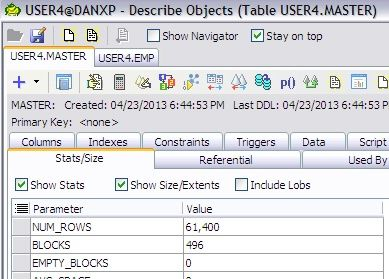This table has 61,400 rows and 496 blocks.

There is an index on the LAST_NAME_TX column. Looking at the column density, this column looks like a good candidate for an index, a very low occurrence of each name across the structure.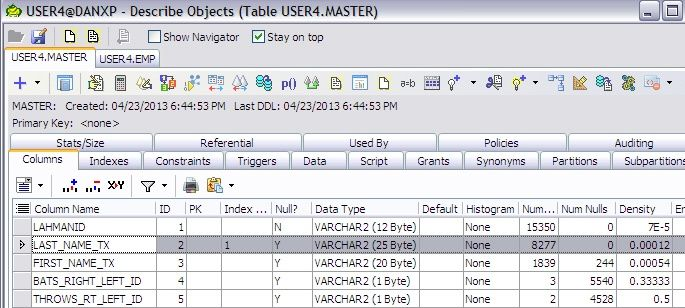Looking at the clustering factor, we see that this number is almost HALF of the total rows in the table!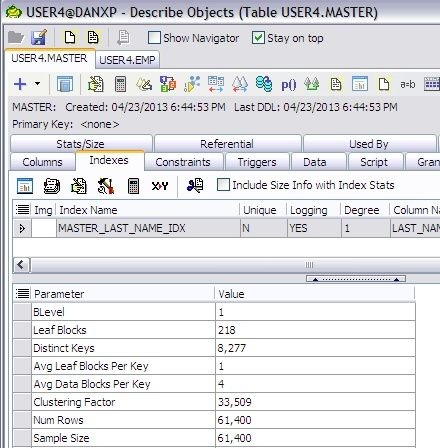At nearly 50% of the number of rows in the table, it is not likely that Oracle would EVER use this index on any kind of a range scan.

TOAD shows important statistics when deciding which columns would be good to index and then again using different statistics, TOAD shows how likely Oracle is to use the index.

Understanding statistics and what they actually mean can shed important light on the indexing of table columns.